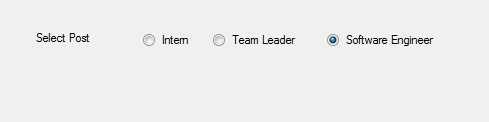# How to set Text in the RadioButton in C#?

• Last Updated : 30 Jun, 2019

In Windows Forms, RadioButton control is used to select a single option among the group of the options. For example, select your gender from the given list, so you will choose only one option among three options like Male or Female or Transgender. In Windows Forms, you are allowed to add text in the RadioButton using the Text Property of the RadioButton which makes your RadioButton meaningful. You can set this property in two different ways:

1. Design-Time: It is the easiest way to add text in the RadioButton as shown in the following steps:

• Step 1: Create a windows form as shown in the below image:
Visual Studio -> File -> New -> Project -> WindowsFormApp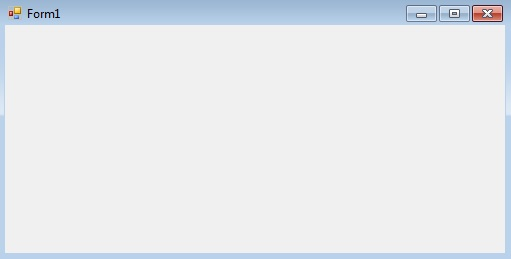• Step 2: Drag the RadioButton control from the ToolBox and drop it on the windows form. You are allowed to place a RadioButton control anywhere on the windows form according to your need.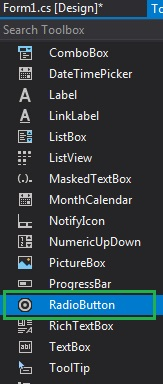• Step 3: After drag and drop you will go to the properties of the RadioButton control to add text in the text of the RadioButton.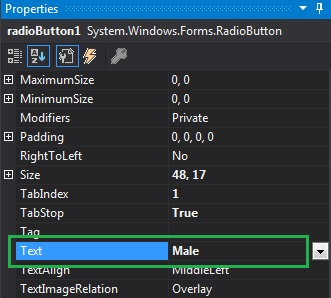Output: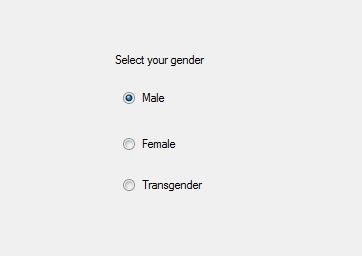2. Run-Time: It is a little bit trickier than the above method. In this method, you can add text in the RadioButton control programmatically with the help of given syntax:

`public override string Text { get; set; }`

The value of this property is of System.String type. The following steps show how to add text in the RadioButton dynamically:

• Step 1: Create a radio button using the RadioButton() constructor is provided by the RadioButton class.
```// Creating radio button
```
• Step 2: After creating RadioButton, set the Text property of the RadioButton provided by the RadioButton class.
```// Adding text in the radio button
r1.Text = "Intern";
```
```// Add this radio button to the form
 `using` `System;``using` `System.Collections.Generic;``using` `System.ComponentModel;``using` `System.Data;``using` `System.Drawing;``using` `System.Linq;``using` `System.Text;``using` `System.Threading.Tasks;``using` `System.Windows.Forms;`` ` `namespace` `WindowsFormsApp23 {`` ` `public` `partial` `class` `Form1 : Form {`` ` `    ``public` `Form1()``    ``{``        ``InitializeComponent();``    ``}`` ` `    ``private` `void` `Form1_Load(``object` `sender, EventArgs e)``    ``{``        ``// Creating and setting label``        ``Label l = ``new` `Label();``        ``l.AutoSize = ``true``;``        ``l.Location = ``new` `Point(176, 40);``        ``l.Text = ``"Select Post"``;`` ` `        ``// Adding this label to the form``        ``this``.Controls.Add(l);`` ` `        ``// Creating and setting the``        ``// properties of the RadioButton``        ``RadioButton r1 = ``new` `RadioButton();``        ``r1.AutoSize = ``true``;``        ``r1.Text = ``"Intern"``;``        ``r1.Location = ``new` `Point(286, 40);``        ``r1.TextAlign = ContentAlignment.MiddleLeft;`` ` `        ``// Adding this label to the form``        ``this``.Controls.Add(r1);`` ` `        ``// Creating and setting the ``        ``// properties of the RadioButton``        ``RadioButton r2 = ``new` `RadioButton();``        ``r2.AutoSize = ``true``;``        ``r2.Text = ``"Team Leader"``;``        ``r2.Location = ``new` `Point(356, 40);``        ``r2.TextAlign = ContentAlignment.MiddleLeft;`` ` `        ``// Adding this label to the form``        ``this``.Controls.Add(r2);`` ` `        ``// Creating and setting the ``        ``// properties of the RadioButton``        ``RadioButton r3 = ``new` `RadioButton();``        ``r3.AutoSize = ``true``;``        ``r3.Text = ``"Software Engineer"``;``        ``r3.Location = ``new` `Point(470, 40);``        ``r3.TextAlign = ContentAlignment.MiddleLeft;`` ` `        ``// Adding this label to the form``        ``this``.Controls.Add(r3);``    ``}``}``}`